# 要刷 LeetCode 了, 才发现自己连时间复杂度都不懂• 时间维度：是指执行当前算法所消耗的时间，我们通常用「时间复杂度」来描述。

• 空间维度：是指执行当前算法需要占用多少内存空间，我们通常用「空间复杂度」来描述。

## 时间复杂度

• 常数阶

• 线性阶

• 平方阶

• 立方阶

• 对数阶

• 线性对数阶

• 指数阶

#### 常数阶

，表示该算法的执行时间（或执行时占用空间）总是为一个常量，不论输入的数据集是大是小，只要是没有循环等复杂结构，那这个代码的时间复杂度就都是O(1)，如：

int i = 1;int j = 2;int k = i + j;

#### 线性阶

，表示一个算法的性能会随着输入数据的大小变化而线性变化，如

for (int i = 0; i < n; i++) {   j = i;   j++;}

#### 平方阶

² 表示一个算法的性能将会随着输入数据的增长而呈现出二次增长。最常见的就是对输入数据进行嵌套循环。如果嵌套层级不断深入的话，算法的性能将会变为立方阶，，以此类推

for(x=1; i<=n; x++){   for(i=1; i<=n; i++){       j = i;       j++;    }}

#### 指数阶

，表示一个算法的性能会随着输入数据的每次增加而增大两倍，典型的方法就是裴波那契数列的递归计算实现

int Fibonacci(int number){    if (number <= 1) return number;    return Fibonacci(number - 2) + Fibonacci(number - 1);}

﻿

#### 对数阶

int i = 1;while(i<n){    i = i * 2;}

#### 线性对数阶

for(m=1; m<n; m++){    i = 1;    while(i<n)    {        i = i * 2;    }}

## 空间复杂度

#### 空间复杂度

int i = 1;int j = 2;++i;j++;int m = i + j;

#### 空间复杂度

int[] m = new int[n]for(i=1; i<=n; ++i){   j = i;   j++;}

## 复杂度速查表

#### 图例#### 大-O 复杂度曲线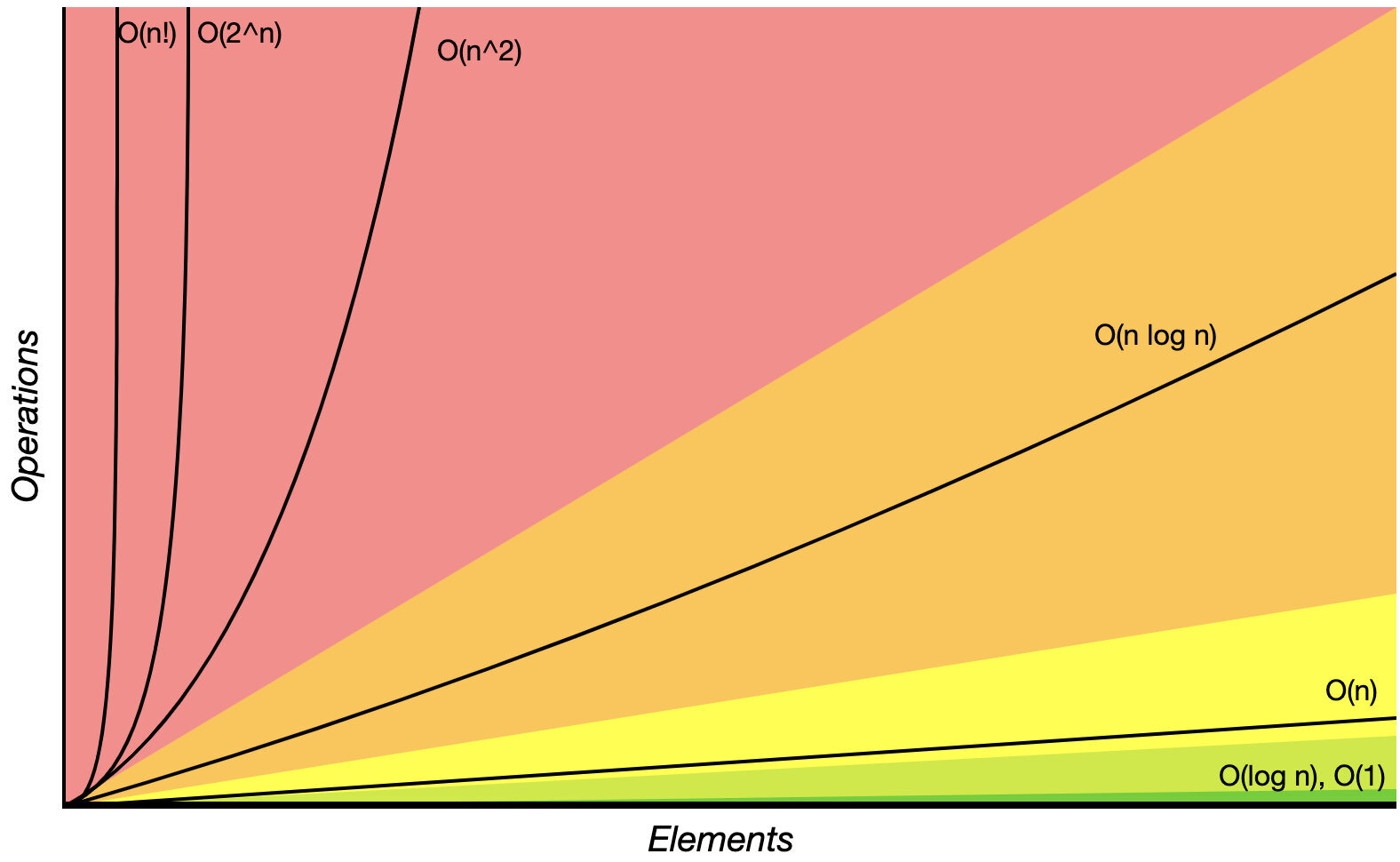#### 抽象数据结构的操作复杂度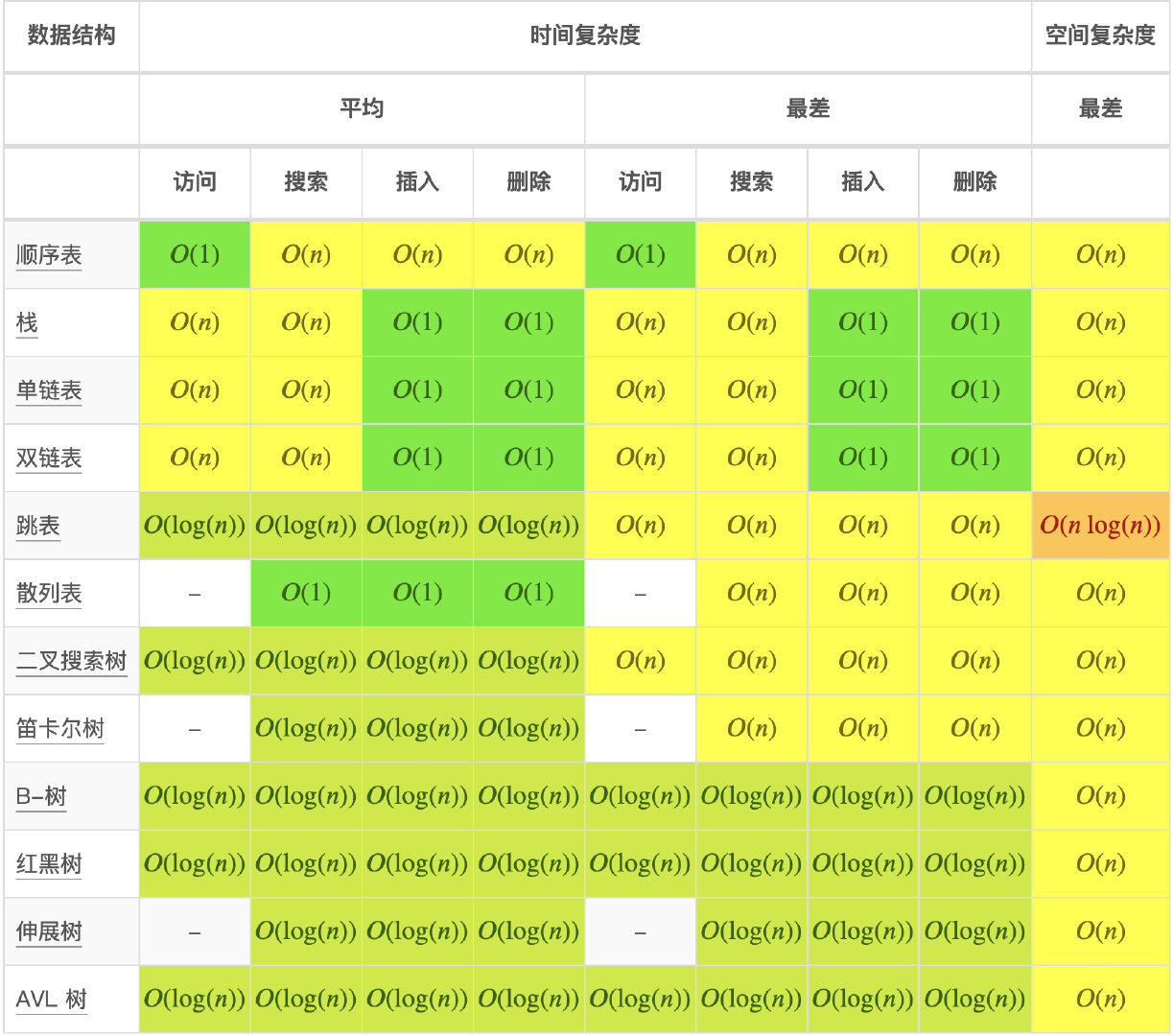#### 数组排序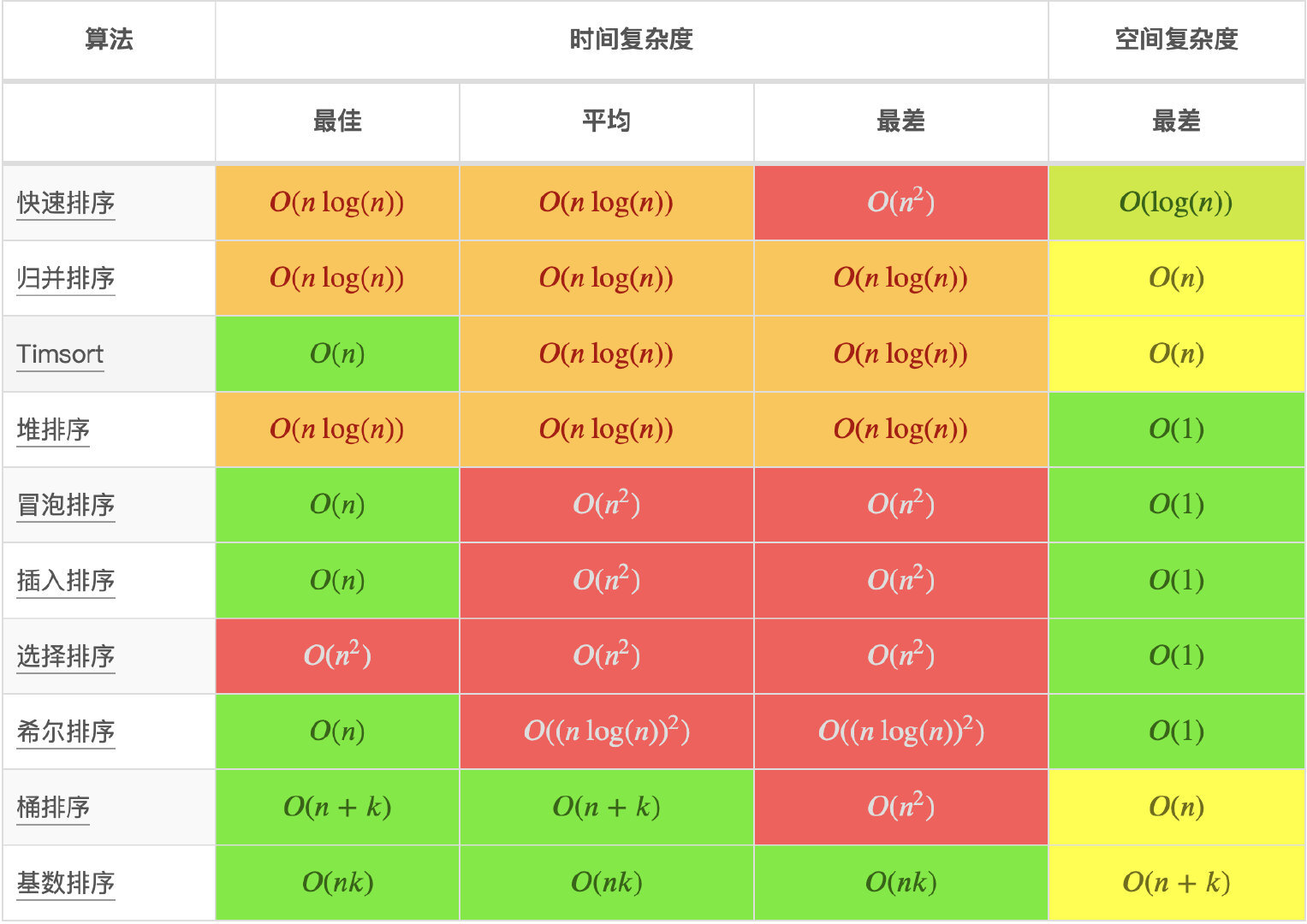#### 图操作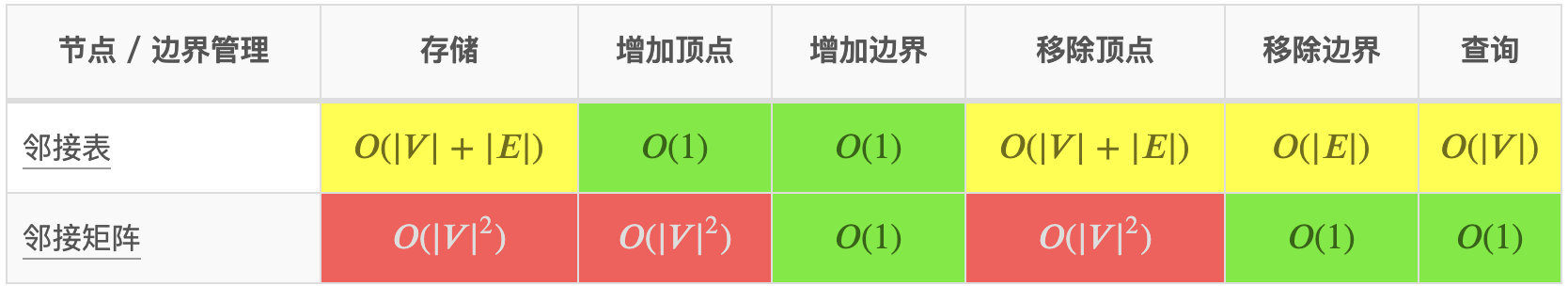#### 堆操作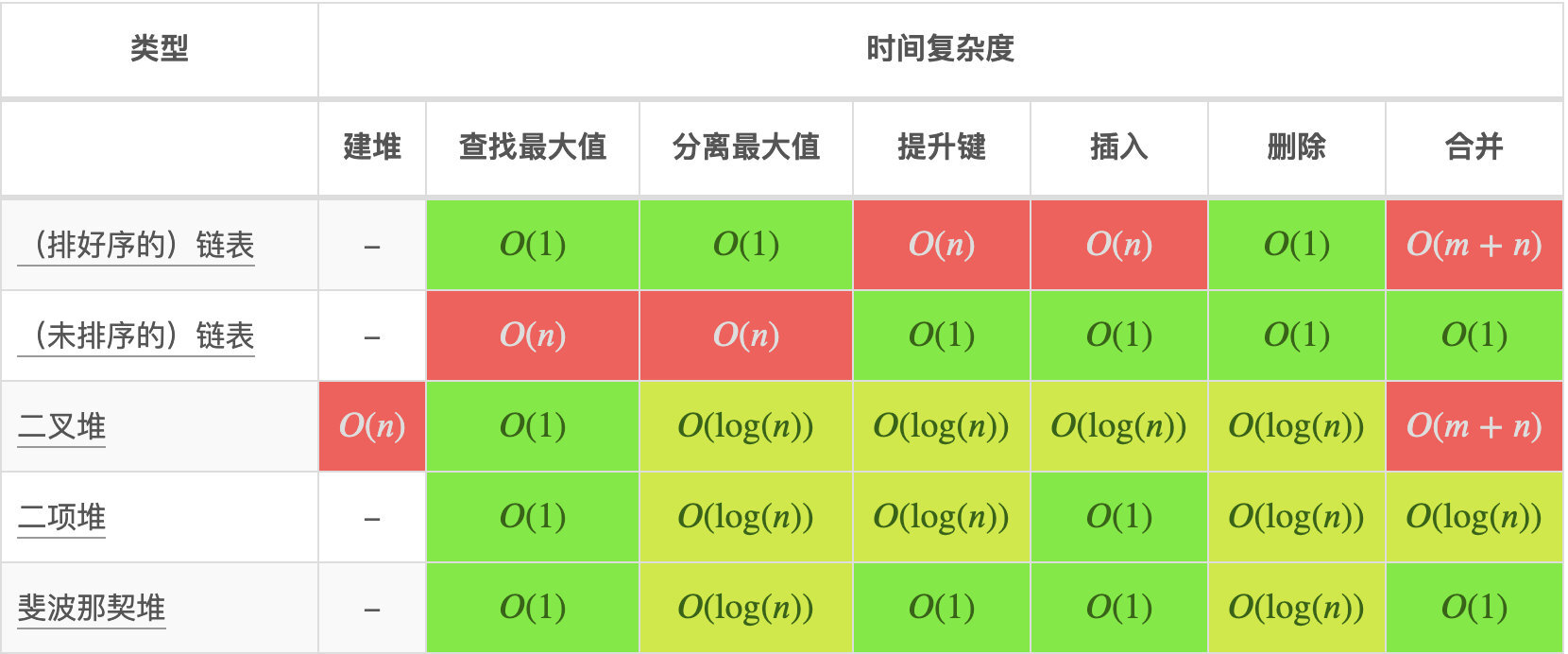## 参考

《大话数据结构》

https://zhuanlan.zhihu.com/p/50479555

﻿### 海星

Javaer成长充电站 2020.02.14 加入

## 评论